Like   Tweet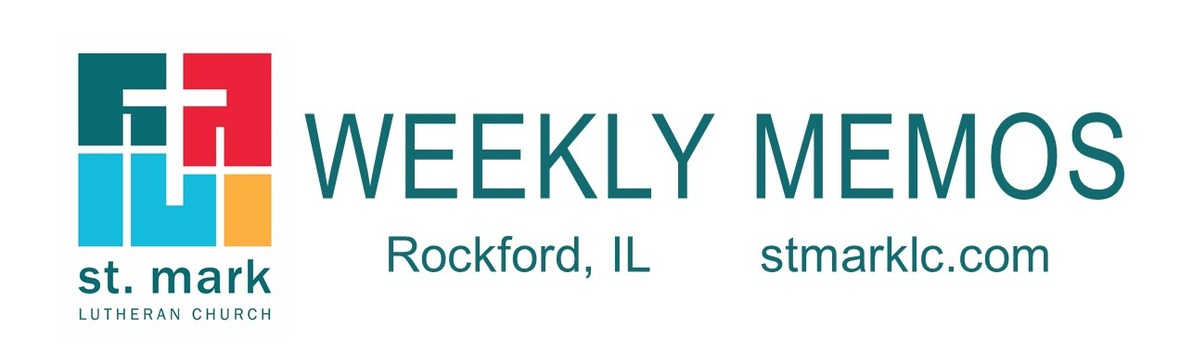table div table+table div table{width:100%;padding:0}table div table+table div table img{width:96.23%;padding:0;float:none}table div table+table div table td{width:100%;padding:0 1.88% 18px}/* styles */## Pastoral ReflectionRemembering Dorothy
Pastor Chad McKenna and Chuck Olson

Today, Pastor Chuck Olson shares his experience caring for a St. Mark member who sadly died this summer. If you or a member of your family are unable to leave home and in need of pastoral care, he is more than eager to connect with you either over the phone or in person. Read more.

## News and Events

 table div table+table+table+table+table+table div table{width:100%;padding:0}table div table+table+table+table+table+table div table img{width:96.23%;padding:0;float:none}table div table+table+table+table+table+table div table td{width:100%;padding:0 1.88% 18px}/* styles *//* styles */ Drive-In Outdoor Worship Service Sunday, October 11 Come join us this Sunday for the 19th Sunday after Pentecost at 9:30 A.M. at St. Mark in the south parking lot. If you are away or unable to go out in public, the service will be live-streamed on our St. Mark YouTube Channel. The link will be emailed to you Sunday morning at 8:00 A.M. and then available for you to watch again and again later in the day, just like our other worship videos. We should mention that for safety reasons our Mulford Road entrance will be closed during these services. When you arrive, you will want to enter via the Featherstone Road entrance. There will be volunteers handing out bulletins and communion wafers. Ushers will guide you to where you need to go. At the end, you can leave either the same way you entered or through the adjoining OSF parking lot along with a bag of donut holes. We can't wait to see you on Sunday, October 11!
 table div table+table+table+table+table+table+table+table div table{width:100%;padding:0}table div table+table+table+table+table+table+table+table div table img{width:96.23%;padding:0;float:none}table div table+table+table+table+table+table+table+table div table td{width:100%;padding:0 1.88% 18px}/* styles */Updated Guidelines for Worship and Small Groups
Due to the new mitigation measures announced for our region, we are tasked with limiting all gatherings to 25 people, both inside and outdoors. To accomplish this, Sunday worshippers will be asked to remain in their vehicles, and the number of musicians will be limited. Those who arrive by bike or foot may sit outside, provided they wear a mask and sit six feet away from others. You will still be able to use the restrooms indoors. Hopefully, these measures can keep the number of people outside a vehicle to 25 or less during worship. In a similar manner, capacity limits during the week for all groups will be reduced to 25 people. We are hopeful that the statistics will improve so that restrictions can ease and our community can continue moving forward!

 table div table+table+table+table+table+table+table+table+table+table div table{width:100%;padding:0}table div table+table+table+table+table+table+table+table+table+table div table img{width:96.23%;padding:0;float:none}table div table+table+table+table+table+table+table+table+table+table div table td{width:100%;padding:0 1.88% 18px}/* styles *//* styles */ Check out a few of the drone videos below. We will be sharing more of the drone images each week.
 table div table+table+table+table+table+table+table+table+table+table+table+table+table+table div table{width:100%;padding:0}table div table+table+table+table+table+table+table+table+table+table+table+table+table+table div table img{width:96.23%;padding:0;float:none}table div table+table+table+table+table+table+table+table+table+table+table+table+table+table div table td{width:100%;padding:0 1.88% 18px}/* styles */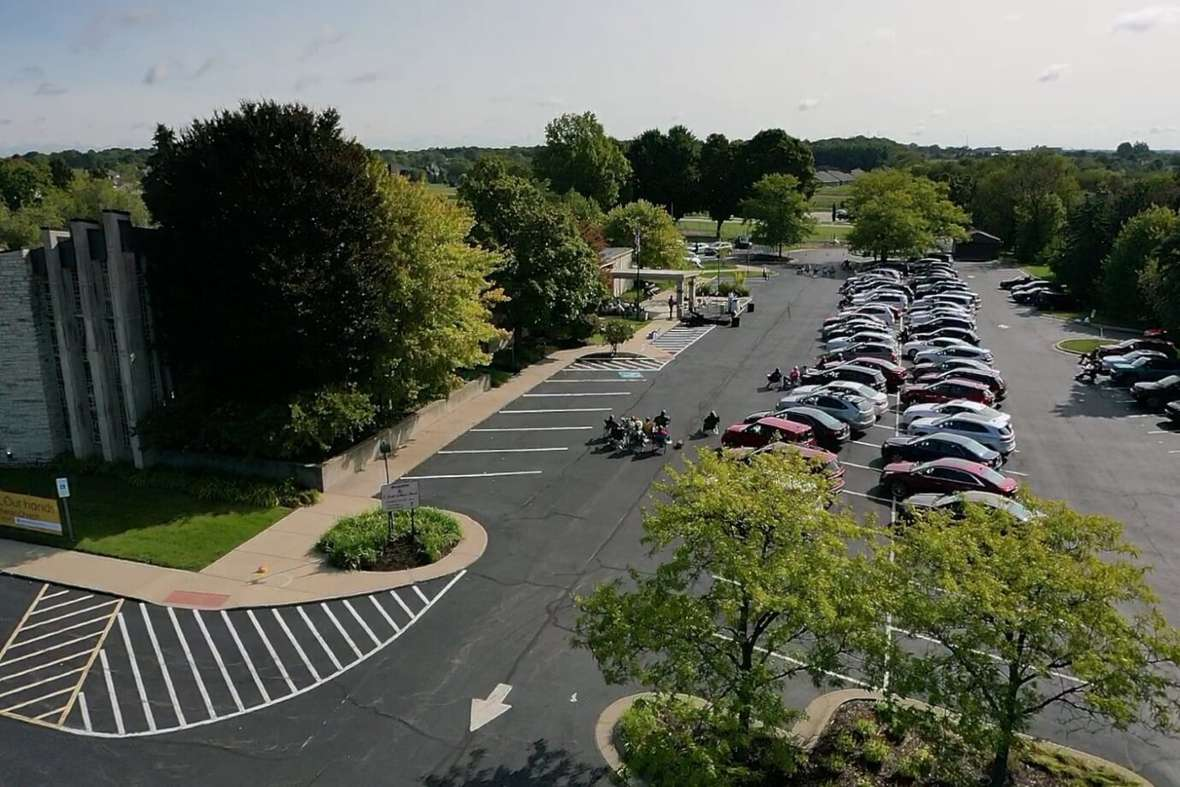table div table+table+table+table+table+table+table+table+table+table+table+table+table+table+table+table div table{width:100%;padding:0}table div table+table+table+table+table+table+table+table+table+table+table+table+table+table+table+table div table img{width:96.23%;padding:0;float:none}table div table+table+table+table+table+table+table+table+table+table+table+table+table+table+table+table div table td{width:100%;padding:0 1.88% 18px}/* styles *//* styles */ God’s Work. Our Hands We will partner with the Northern Illinois Food Bank, St. Mark, and the youth ministry team to host a Mobile food pantry at St. Mark on three occasions this fall: Wednesday, Oct 21; Saturday, November 21; and Saturday, December 19. Volunteer commitment time would be from 10:30 A.M.-1:00 P.M. The food bank will bring food for 250 families. Everyone is welcome! We need volunteers for each of those days. Please consider joining team October, November or December. We will be loading boxes of groceries into trunks of cars as they drive through our parking lot. Please call or text 815-871-0390 or call the church office to sign up. You may also email melinda@stmarklc.com. Invite your friends, family, co-workers, teammates and classmates. This is a socially distanced event. We will be wearing masks and gloves! “Many hands make for light work.”
 table div table+table+table+table+table+table+table+table+table+table+table+table+table+table+table+table+table+table+table div table{width:100%;padding:0}table div table+table+table+table+table+table+table+table+table+table+table+table+table+table+table+table+table+table+table div table img{width:96.23%;padding:0;float:none}table div table+table+table+table+table+table+table+table+table+table+table+table+table+table+table+table+table+table+table div table td{width:100%;padding:0 1.88% 18px}/* styles */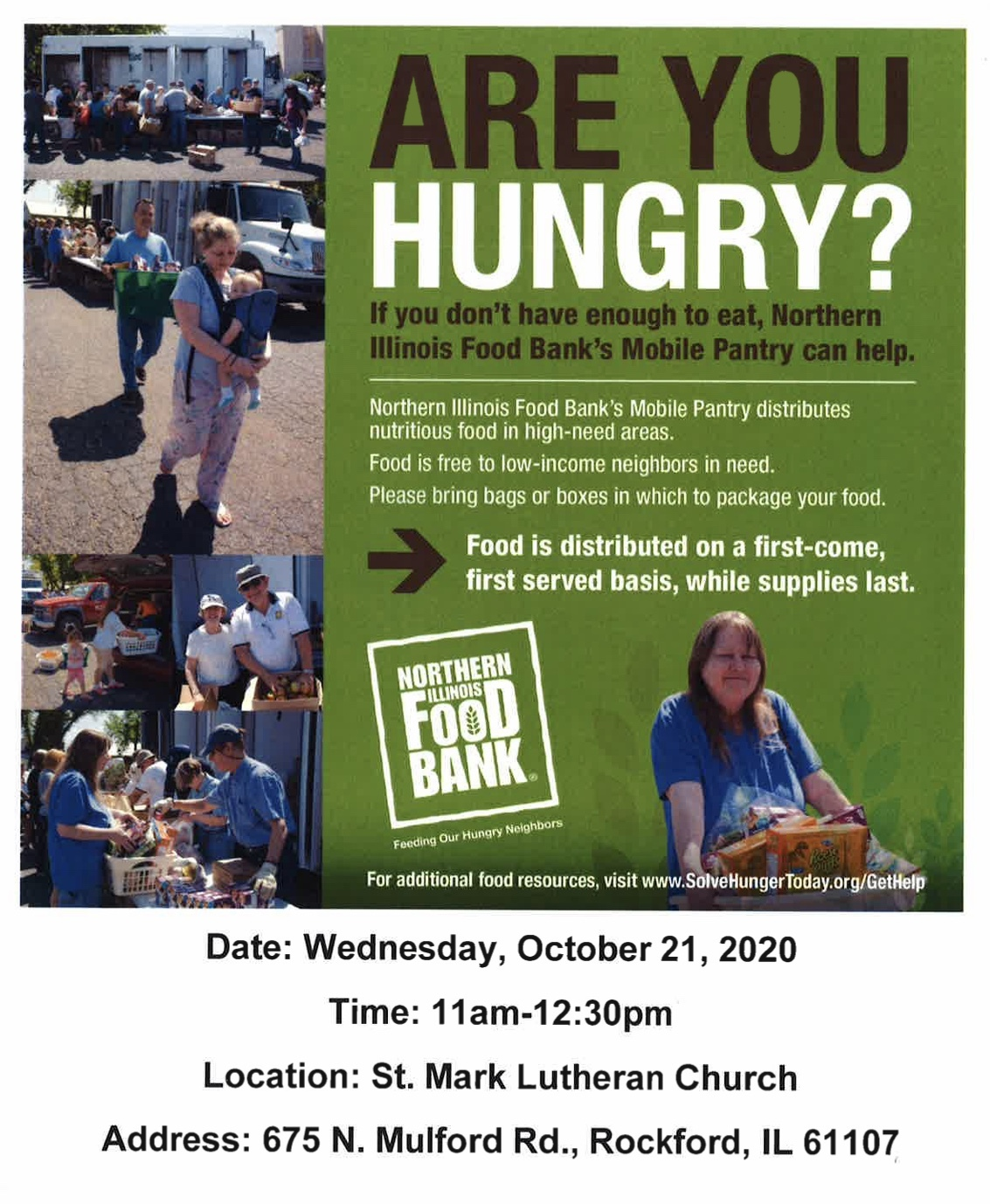table div table+table+table+table+table+table+table+table+table+table+table+table+table+table+table+table+table+table+table+table+table div table{width:100%;padding:0}table div table+table+table+table+table+table+table+table+table+table+table+table+table+table+table+table+table+table+table+table+table div table img{width:96.23%;padding:0;float:none}table div table+table+table+table+table+table+table+table+table+table+table+table+table+table+table+table+table+table+table+table+table div table td{width:100%;padding:0 1.88% 18px}/* styles */Save the Date:
We will be having a blood drive on Tuesday, November 24 from 1:30 -7:30 P.M. More information will be coming soon.

 table div table+table+table+table+table+table+table+table+table+table+table+table+table+table+table+table+table+table+table+table+table+table+table div table{width:100%;padding:0}table div table+table+table+table+table+table+table+table+table+table+table+table+table+table+table+table+table+table+table+table+table+table+table div table img{width:96.23%;padding:0;float:none}table div table+table+table+table+table+table+table+table+table+table+table+table+table+table+table+table+table+table+table+table+table+table+table div table td{width:100%;padding:0 1.88% 18px}/* styles */CARE Package addresses are due by October 15th. Parents, please send addresses for St. Mark college and military members. We want to send words of encouragement but first, we need their address. Please e-mail melinda@stmarklc.com or call the church office. 815-398-3557. Thank you!

 table div table+table+table+table+table+table+table+table+table+table+table+table+table+table+table+table+table+table+table+table+table+table+table+table+table div table{width:100%;padding:0}table div table+table+table+table+table+table+table+table+table+table+table+table+table+table+table+table+table+table+table+table+table+table+table+table+table div table img{width:96.23%;padding:0;float:none}table div table+table+table+table+table+table+table+table+table+table+table+table+table+table+table+table+table+table+table+table+table+table+table+table+table div table td{width:100%;padding:0 1.88% 18px}/* styles */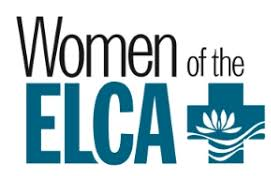Miriam Circle
We are happy to announce that the Miriam Circle will resume meeting on October 14 at 1:00 PM in the Common Grounds Cafe. Carole Malmgren will lead our devotions.

All ladies are welcome to attend as we gather in fellowship, prayer, and support of one another. Please contact one of the hostesses Karen Clements at 815 398-2657 or Betty Sparks at 815 636-1122 or email kbsp4968@msn.com if you plan to attend. The guidelines for CO-VD19 will be observed.

 table div table+table+table+table+table+table+table+table+table+table+table+table+table+table+table+table+table+table+table+table+table+table+table+table+table+table+table div table{width:100%;padding:0}table div table+table+table+table+table+table+table+table+table+table+table+table+table+table+table+table+table+table+table+table+table+table+table+table+table+table+table div table img{width:96.23%;padding:0;float:none}table div table+table+table+table+table+table+table+table+table+table+table+table+table+table+table+table+table+table+table+table+table+table+table+table+table+table+table div table td{width:100%;padding:0 1.88% 18px}/* styles */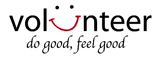Interested in helping out on Sunday mornings?
We are looking for someone to help during the 9:30 outdoor drive-in worship service with the sound. No prior experience necessary. Your job would be to run one microphone volume, iPad volume, master volume and play 2-3 tracks off the iPad. The band sets up their microphones. You would not have to commit to every Sunday. Please be at church by 9:00 am to get a quick tour of it all. Email Jill Davenport at jill@stmarklc.com or call the church office 815-398-3557. Thank you.

 table div table+table+table+table+table+table+table+table+table+table+table+table+table+table+table+table+table+table+table+table+table+table+table+table+table+table+table+table+table div table{width:100%;padding:0}table div table+table+table+table+table+table+table+table+table+table+table+table+table+table+table+table+table+table+table+table+table+table+table+table+table+table+table+table+table div table img{width:96.23%;padding:0;float:none}table div table+table+table+table+table+table+table+table+table+table+table+table+table+table+table+table+table+table+table+table+table+table+table+table+table+table+table+table+table div table td{width:100%;padding:0 1.88% 18px}/* styles */Volunteer Zoom Moderator Needed!
Sunday school is in need of a Zoom Moderator for our online classes! This is technical support - not teaching. This volunteer will admit families to the virtual classroom, help manage the group’s audio, watch the chat, and help with whatever other technical problems pop up along the way. I would love to have one dedicated volunteer who can help every Sunday, but if that’s not possible it can probably be a shared job between two people.

If you are a student who needs volunteer hours, this is a great way to earn them without leaving home!

Email julie@stmarklc.com with questions or to volunteer! Thank you!

 table div table+table+table+table+table+table+table+table+table+table+table+table+table+table+table+table+table+table+table+table+table+table+table+table+table+table+table+table+table+table+table div table{width:100%;padding:0}table div table+table+table+table+table+table+table+table+table+table+table+table+table+table+table+table+table+table+table+table+table+table+table+table+table+table+table+table+table+table+table div table img{width:96.23%;padding:0;float:none}table div table+table+table+table+table+table+table+table+table+table+table+table+table+table+table+table+table+table+table+table+table+table+table+table+table+table+table+table+table+table+table div table td{width:100%;padding:0 1.88% 18px}/* styles */St. Mark Mobile App!

This is different than our church directory app.
Our mobile app is designed specifically for St. Mark to improve our communication with our congregation and nurture our members. We encourage you to download the app. It's about getting involved and staying connected.

 table div table+table+table+table+table+table+table+table+table+table+table+table+table+table+table+table+table+table+table+table+table+table+table+table+table+table+table+table+table+table+table+table+table div table{width:100%;padding:0}table div table+table+table+table+table+table+table+table+table+table+table+table+table+table+table+table+table+table+table+table+table+table+table+table+table+table+table+table+table+table+table+table+table div table img{width:96.23%;padding:0;float:none}table div table+table+table+table+table+table+table+table+table+table+table+table+table+table+table+table+table+table+table+table+table+table+table+table+table+table+table+table+table+table+table+table+table div table td{width:100%;padding:0 1.88% 18px}/* styles *//* styles */ Masks are still available! There is a bin of masks, outside the south doors. Monday, Wednesday, and Friday from 10:00 A.M. till Noon. If you need masks dropped to your residence, children size, or questions text/call Melinda Alekna 815-871-0390. If you would like to help with delivering to people, we could use your help as well. Thank you to St. Mark “sewers” for making 500 masks. The masks are being donated to the ELCA 75,000 mask challenge. Masks will be sent to third world countries in need.
 table div table+table+table+table+table+table+table+table+table+table+table+table+table+table+table+table+table+table+table+table+table+table+table+table+table+table+table+table+table+table+table+table+table+table+table div table{width:100%;padding:0}table div table+table+table+table+table+table+table+table+table+table+table+table+table+table+table+table+table+table+table+table+table+table+table+table+table+table+table+table+table+table+table+table+table+table+table div table img{width:96.23%;padding:0;float:none}table div table+table+table+table+table+table+table+table+table+table+table+table+table+table+table+table+table+table+table+table+table+table+table+table+table+table+table+table+table+table+table+table+table+table+table div table td{width:100%;padding:0 1.88% 18px}/* styles */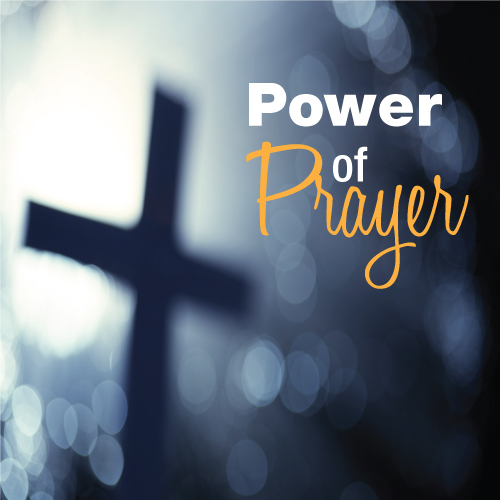St. Mark Prayer Chain
Would you like to be a part of the St. Mark prayer chain? Prayer requests come into the church office weekly. As we receive prayer requests we would send out an e-mail and ask that you pray daily for the persons mentioned in the requests. All requests are to remain confidential. Please e-mail melinda@stmarklc.com if you would like to participate in the prayer chain. “Be anxious for nothing, but in everything by prayer and supplication, with thanksgiving, let your requests be made known to God.” Colossians 4:2.

 table div table+table+table+table+table+table+table+table+table+table+table+table+table+table+table+table+table+table+table+table+table+table+table+table+table+table+table+table+table+table+table+table+table+table+table+table+table div table{width:100%;padding:0}table div table+table+table+table+table+table+table+table+table+table+table+table+table+table+table+table+table+table+table+table+table+table+table+table+table+table+table+table+table+table+table+table+table+table+table+table+table div table img{width:96.23%;padding:0;float:none}table div table+table+table+table+table+table+table+table+table+table+table+table+table+table+table+table+table+table+table+table+table+table+table+table+table+table+table+table+table+table+table+table+table+table+table+table+table div table td{width:100%;padding:0 1.88% 18px}/* styles */Instant Church Directory
This is a great time to stay connected with our St. Mark family. If you need help downloading the app or creating an account on your computer or ipad, please call Jill Davenport at 815-218-3403 or email jill@stmarklc.com and she will be happy to help you through the process.

Instant Church Directory

Mobile App Login Instructions for Android & Kindle Devices

We also have a form for you to complete to make sure we have the most current information for the directory. There are paper forms at church or click on the link to Church Directory Form

 table div table+table+table+table+table+table+table+table+table+table+table+table+table+table+table+table+table+table+table+table+table+table+table+table+table+table+table+table+table+table+table+table+table+table+table+table+table+table+table div table{width:100%;padding:0}table div table+table+table+table+table+table+table+table+table+table+table+table+table+table+table+table+table+table+table+table+table+table+table+table+table+table+table+table+table+table+table+table+table+table+table+table+table+table+table div table img{width:96.23%;padding:0;float:none}table div table+table+table+table+table+table+table+table+table+table+table+table+table+table+table+table+table+table+table+table+table+table+table+table+table+table+table+table+table+table+table+table+table+table+table+table+table+table+table div table td{width:100%;padding:0 1.88% 18px}/* styles */Office Hours
Monday through Friday 9:00 A.M. - 1:00 P.M. As always, please call the office or Pastor Chad if you have any needs or concerns.

 table div table+table+table+table+table+table+table+table+table+table+table+table+table+table+table+table+table+table+table+table+table+table+table+table+table+table+table+table+table+table+table+table+table+table+table+table+table+table+table+table+table div table{width:100%;padding:0}table div table+table+table+table+table+table+table+table+table+table+table+table+table+table+table+table+table+table+table+table+table+table+table+table+table+table+table+table+table+table+table+table+table+table+table+table+table+table+table+table+table div table img{width:96.23%;padding:0;float:none}table div table+table+table+table+table+table+table+table+table+table+table+table+table+table+table+table+table+table+table+table+table+table+table+table+table+table+table+table+table+table+table+table+table+table+table+table+table+table+table+table+table div table td{width:100%;padding:0 1.88% 18px}/* styles */## Children's MinistryRemote Sunday School
Our online Sunday school classes have begun. If you haven't registered yet, please email Julie at julie@stmarklc.com.

We meet at 11:00 a.m. on Sundays. If the service runs a little long, we’ll adjust. See you on Sunday!

 table div table+table+table+table+table+table+table+table+table+table+table+table+table+table+table+table+table+table+table+table+table+table+table+table+table+table+table+table+table+table+table+table+table+table+table+table+table+table+table+table+table+table+table+table div table{width:100%;padding:0}table div table+table+table+table+table+table+table+table+table+table+table+table+table+table+table+table+table+table+table+table+table+table+table+table+table+table+table+table+table+table+table+table+table+table+table+table+table+table+table+table+table+table+table+table div table img{width:96.23%;padding:0;float:none}table div table+table+table+table+table+table+table+table+table+table+table+table+table+table+table+table+table+table+table+table+table+table+table+table+table+table+table+table+table+table+table+table+table+table+table+table+table+table+table+table+table+table+table+table div table td{width:100%;padding:0 1.88% 18px}/* styles */## Outreach Ministry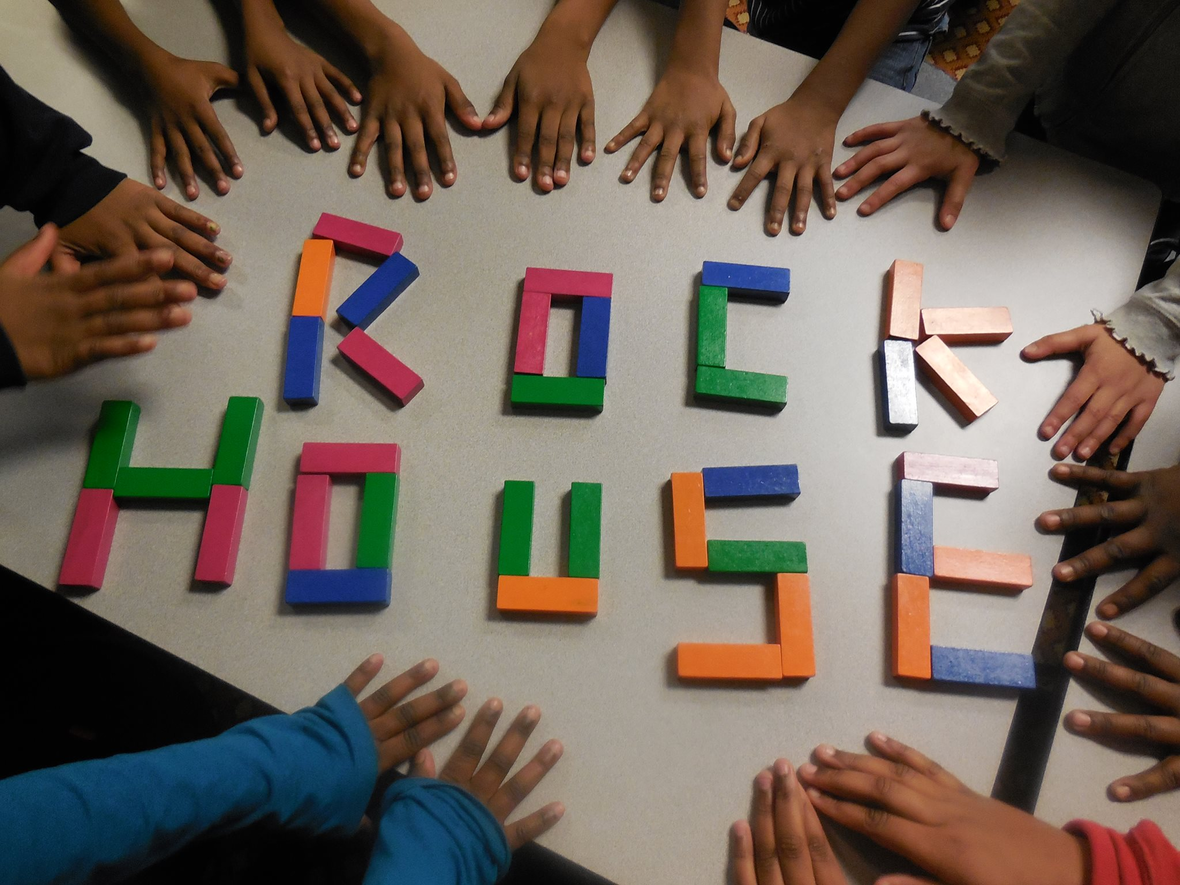The Outreach Committee is continuing to serve one meal a month for Rock House Kids. Volunteers are needed to prepare food for 75 children in grades 1st through 6th on the following dates: October 21st, November 4th and December 2nd. If interested please call Beth or John Johnson at 815-332-4570 for menu ideas and other details about the program.

 table div table+table+table+table+table+table+table+table+table+table+table+table+table+table+table+table+table+table+table+table+table+table+table+table+table+table+table+table+table+table+table+table+table+table+table+table+table+table+table+table+table+table+table+table+table+table+table div table{width:100%;padding:0}table div table+table+table+table+table+table+table+table+table+table+table+table+table+table+table+table+table+table+table+table+table+table+table+table+table+table+table+table+table+table+table+table+table+table+table+table+table+table+table+table+table+table+table+table+table+table+table div table img{width:96.23%;padding:0;float:none}table div table+table+table+table+table+table+table+table+table+table+table+table+table+table+table+table+table+table+table+table+table+table+table+table+table+table+table+table+table+table+table+table+table+table+table+table+table+table+table+table+table+table+table+table+table+table+table div table td{width:100%;padding:0 1.88% 18px}/* styles */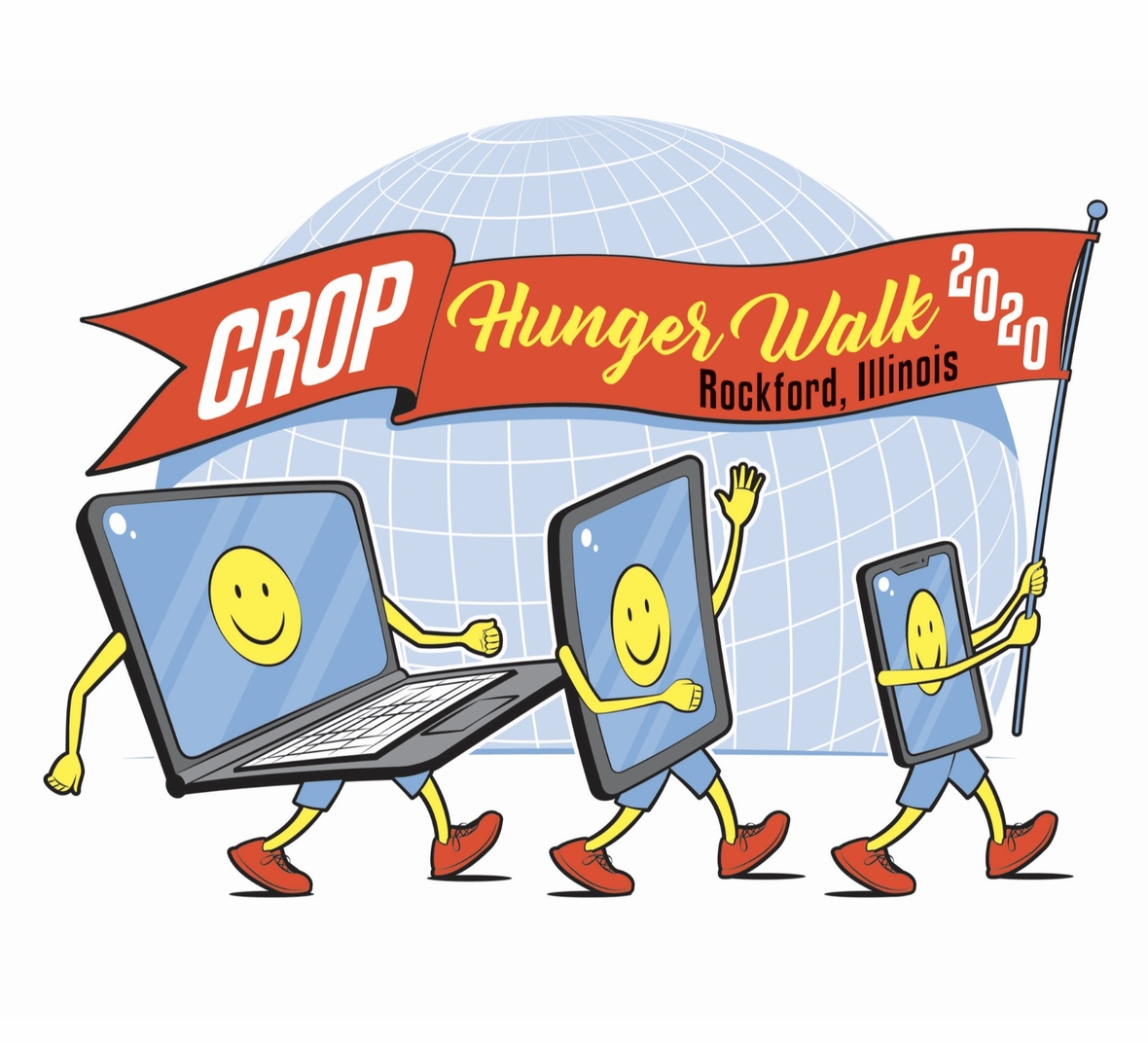CROP Walk Goes Virtual
This year the Crop Walk is going virtual. Please consider a donation on the St. Mark app, website, or a check to St. Mark.

Please read this Rockford Register Star letter to the editor. It expresses the urgent need for our CROP Hunger Walk. Its writer is the CEO of the Northern Illinois Food Bank.

LETTERS TO THE EDITOR
Congress must extend food-program waivers
Hunger doesn’t wait — for the next paycheck, the next stimulus bill, or for the end of a global pandemic. Our work to solve hunger has never been more urgent — and we need the support of our elected officials to make it happen.

Our neighbors need our help now more than ever. Northern Illinois Food Bank continues to see a 30%50% increase in families seeking assistance from our network, many for the first time. The U.S. Department of Agriculture has taken steps to keep families fed, including waiver flexibility for family and child nutrition programs including SNAP, WIC, SFSP and CACFP. These waivers allow for remote services, extended operations, and additional meals to be served, all while maintaining vital safety practices. However, while many of these waivers are sunsetting soon, we know food insecurity will not.

We need members of Congress to continue advocating for our neighbors throughout this crisis, by urging the USDA to extend these
waivers for the duration of the pandemic. In doing so, together, we can help keep food on the table for some of our most vulnerable neighbors during this season of unprecedented and prolonged uncertainty.
— Julie Yurko, president and CEO, Northern Illinois Food Bank

 table div table+table+table+table+table+table+table+table+table+table+table+table+table+table+table+table+table+table+table+table+table+table+table+table+table+table+table+table+table+table+table+table+table+table+table+table+table+table+table+table+table+table+table+table+table+table+table+table+table div table{width:100%;padding:0}table div table+table+table+table+table+table+table+table+table+table+table+table+table+table+table+table+table+table+table+table+table+table+table+table+table+table+table+table+table+table+table+table+table+table+table+table+table+table+table+table+table+table+table+table+table+table+table+table+table div table img{width:96.23%;padding:0;float:none}table div table+table+table+table+table+table+table+table+table+table+table+table+table+table+table+table+table+table+table+table+table+table+table+table+table+table+table+table+table+table+table+table+table+table+table+table+table+table+table+table+table+table+table+table+table+table+table+table+table div table td{width:100%;padding:0 1.88% 18px}/* styles */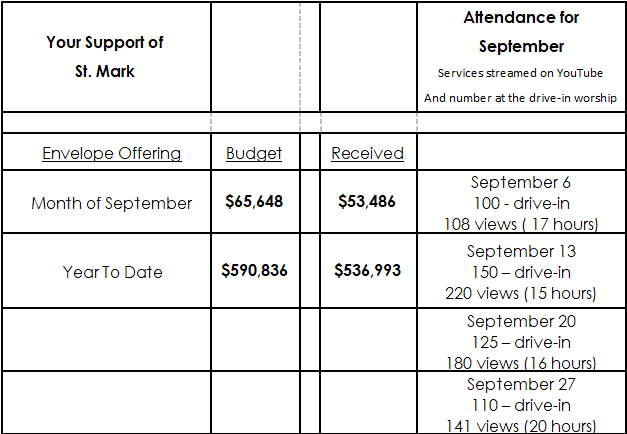table div table+table+table+table+table+table+table+table+table+table+table+table+table+table+table+table+table+table+table+table+table+table+table+table+table+table+table+table+table+table+table+table+table+table+table+table+table+table+table+table+table+table+table+table+table+table+table+table+table+table+table div table{width:100%;padding:0}table div table+table+table+table+table+table+table+table+table+table+table+table+table+table+table+table+table+table+table+table+table+table+table+table+table+table+table+table+table+table+table+table+table+table+table+table+table+table+table+table+table+table+table+table+table+table+table+table+table+table+table div table img{width:96.23%;padding:0;float:none}table div table+table+table+table+table+table+table+table+table+table+table+table+table+table+table+table+table+table+table+table+table+table+table+table+table+table+table+table+table+table+table+table+table+table+table+table+table+table+table+table+table+table+table+table+table+table+table+table+table+table+table div table td{width:100%;padding:0 1.88% 18px}/* styles */## Our St. Mark members:

Rose Pearson, Betty Adams, Nancy Scheel, Billie Reinhold, Jim Stewart, Constance Carlson, Marilyn Vivian, Sherri Lindquist, Tim Vivian, Bill Jennings, Tim Panjkovich, Bette Patterson, Jerry Cazaniga, David Young, Keith Haggestad.

## Family and friends of St. Mark members:

Butch Cotter, Wanda Adamson, Denise Nichols, Milane Bagel, Gayle Coffler, Darold Beekmann, Janet Webster, Rae Ann McIntire, Linda Johnson, Robert and Cindy Wilson, Pastor Jane McChesney, Emily Tropp, Louis Fivaz, Mike Leonard, Katelynn Audette, Eva Love Sherbondy, Norma Comstock, Carolyn King, Ashton Henley, Nathan Gyllin.

## Those serving in the military and their families:

Hunter Haggestad, Tristan Davenport, Scot Hornick, Bailey Panjkovich, Xander Gehrke, Jim Tammen, Brandon Ballenger, Taylor Eversole, Dean Barron.

Strengthen and encourage those in public health services and in the medical profession: care-givers, nurses, attendants, doctors, all who commit themselves to care for the sick and their families.

 table div table+table+table+table+table+table+table+table+table+table+table+table+table+table+table+table+table+table+table+table+table+table+table+table+table+table+table+table+table+table+table+table+table+table+table+table+table+table+table+table+table+table+table+table+table+table+table+table+table+table+table+table+table+table div table{width:100%;padding:0}table div table+table+table+table+table+table+table+table+table+table+table+table+table+table+table+table+table+table+table+table+table+table+table+table+table+table+table+table+table+table+table+table+table+table+table+table+table+table+table+table+table+table+table+table+table+table+table+table+table+table+table+table+table+table div table img{width:96.23%;padding:0;float:none}table div table+table+table+table+table+table+table+table+table+table+table+table+table+table+table+table+table+table+table+table+table+table+table+table+table+table+table+table+table+table+table+table+table+table+table+table+table+table+table+table+table+table+table+table+table+table+table+table+table+table+table+table+table+table div table td{width:100%;padding:0 1.88% 18px}/* styles *//* styles */ Office Hours Monday through Friday 9:00 A.M. - 1:00 P.M. Telephone: 815-398-3557 Publications St. Mark Memos, the weekly e-newsletter, is distributed each Thursday. The deadline for submission is Wednesday by 4:00 P.M. Please click to send submissions. Sermons Online Miss a Sunday at St. Mark? Please visit our website and watch the sermons online. Please click to watch.